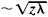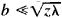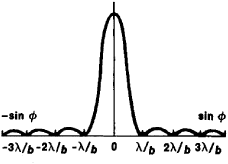# Diffraction of Light

Also found in: Dictionary, Thesaurus, Medical.
Related to Diffraction of Light: Polarization of light
The following article is from The Great Soviet Encyclopedia (1979). It might be outdated or ideologically biased.

## Diffraction of Light

a phenomena observed in cases of the propagation of light past the sharp edges of opaque or transparent bodies or through narrow apertures. In such cases the rectilinearity of the propagation of light is disrupted—that is, a deviation from the laws of geometrical optics occurs. As a result of the diffraction of light, when an opaque screen is illuminated by a point source of light a number of light and dark diffraction fringes are observed at the border of the shadow, where, according to the laws of geometrical optics, an abrupt jump from shadow to light should occur. Since diffraction is inherent in any wave motion, the discovery of the diffraction of light by the Italian physicist and astronomer F. Grimaldi in the 17th century and its explanation by the French physicist A. Fresnel in the early 19th century were among the fundamental proofs of the wave nature of light.

The approximation theory of the diffraction of light is based on an application of the Huygens-Fresnel principle. The construction of Fresnel zones may be used for qualitative consideration of the simplest cases of light diffraction. As light passes from a point source through a small round aperture in an opaque screen or around a small opaque screen, diffraction fringes are observed in the form of concentratic circles. If the aperture leaves open an even number of zones, then a dark spot results at the center of the diffraction patterns, and when the number of zones is odd, a light spot appears. A light spot results at the center of the shadow from a round screen that covers a not very large number of Fresnel zones.

Two cases of the diffraction of light are distinguished: the diffraction of a spherical wave, in which the size of the aperture is comparable to the size of the Fresnel zone—that is, b, where b is the size of the aperture, z is the distance of the observation point from the screen, and λ is the wavelength (Fresnel diffraction); and the diffraction of light in parallel beams, in which the aperture is much smaller than one Fresnel zone—that is,(Fraunhofer diffraction). In the latter case, when a parallel beam of light is incident on the aperture the beam becomes divergent, with an angle φ ~ λ/b (diffraction divergence).

The case of the diffraction of light by a slit is of great practical importance. Upon illumination of a slit by a parallel beam of monochromatic light, a number of dark and light bands or fringes that attenuate rapidly in intensity results on the screen. If the light is perpendicular to the plane of the slit, then the fringes are situated symmetrically with respect to the central fringe (Figure 1), and the illumination changes along the screen periodically with the change in φ, becoming 0 at angles φ for which sin φ = mλ/b (m = 1, 2, 3, …). At intermediate values the illumination reaches maximum values. The main maximum occurs when m = 0, where sin φ = 0, that is, φ = 0 . The subsequent maximums, which are much smaller than the main maximum, correspond to the values of φ determined from the conditions sin φ = 1.43λ/b, 2.46λ/b, 3.47λ/b, and so on.Figure 1. Fraunhofer diffraction by a slit

As the width of the slit decreases, the central light fringe expands, and at a certain width the position of the minimums and maximums depends on X—that is, the greater X, the greater the distance between the fringes. Therefore, in the case of white light the aggregate of the respective patterns for various colors occurs. Here the main maximum will be common to all × and can be represented in the form of a white band that gives way to colored bands with alternation of colors from violet to red.

If there are two identical parallel slits, they yield identical diffraction patterns that are superimposed on each other, as a result of which the maximums are correspondingly increased; in addition, interference of the waves from the first and second slits takes place, which greatly complicates the pattern. As a result the minimums will be at their previous places, since these are the directions in which neither slit is sending out light. Moreover, directions are possible in which the light beams sent out by the two slits cancel each other. Thus, the former minimums are determined by the conditions b sin φ = λ, 2λ, 3λ, … ; additional minimums are d sin φ = A/2, 3λ/2, 5λ/2, … (d is the size of slit b together with the opaque interval a); and the main maximums are d sin φ = 0, λ, 2λ, 3λ, … (that is, one additional minimum is located between the two main maximums, but the maximums become narrower than when one slit is present). An increase in the number of slits makes this phenomenon even more pronounced.

The diffraction of light plays a significant role in the scattering of light in turbid mediums such as dust particles and droplets of fog. The operation of spectral devices with a diffraction grid (diffraction spectrometers) is based on the diffraction of light. The diffraction of light determines the limit of the resolution of optical instruments (telescopes and microscopes). As a result of the diffraction of light the image of a point source (such as stars in a telescope) has the form of a circle with a diameter λf/D, where D is the diameter of the lens and/is its focal length. The divergence of laser radiation is also determined by the diffraction of light. To reduce the divergence of a laser beam it is transformed into a broader beam by means of a telescope, and then the divergence of the radiation is determined by the diameter D of the lens according to the formula φ~λ/D.

### REFERENCES

Landsberg, G. S. Optika, 4th ed. Moscow, 1957. (Obshchii kurs fiziki, vol. 3.)
Gorelik, G. S. Kolebaniia i volny, 2nd ed. Moscow, 1959. Chapter 9.
The Great Soviet Encyclopedia, 3rd Edition (1970-1979). © 2010 The Gale Group, Inc. All rights reserved.
References in periodicals archive ?
The first mathematical theory of diffraction was derived in Fresnel's 1819 Memoir on the Diffraction of Light (1) from a surprisingly minimal set of assumptions:
All generated diffractive images that shared similar visual properties and effects that worked on the diffraction of light, even if the origination techniques used were not necessarily based on interference.
Indigo buntings have no blue pigment; they are actually black, but the diffraction of light through the structure of the feathers makes them appear blue.
explained, "Indigo buntings have no pigment; they are actually black, but the diffraction of light through the structure of the feathers makes them appear blue." (In winter, the male loses much of his blue sheen and looks more like the plain brown female.)
(Interestingly, the colors we sometimes see on roads after a rain are formed by the diffraction of light from incredibly thin deposits of oil on the road surfaces.)

Site: Follow: Share:
Open / Close